# Chemistry- CY 2013 GATE Paper (Practice Test)

## 65 Questions MCQ Test GATE Past Year Papers for Practice (All Branches) | Chemistry- CY 2013 GATE Paper (Practice Test)

Description
Attempt Chemistry- CY 2013 GATE Paper (Practice Test) | 65 questions in 180 minutes | Mock test for GATE preparation | Free important questions MCQ to study GATE Past Year Papers for Practice (All Branches) for GATE Exam | Download free PDF with solutions
QUESTION: 1

Solution:
QUESTION: 2

Solution:
QUESTION: 3

### Mahatama Gandhi was known for his humility as

Solution:
QUESTION: 4

"All engineering students" "should learn mechanics", "mathematics and"  "how to do computation"

I                                             II                                    III                                IV

Which of the above underlined parts of the sentence is not appropriate?

Solution:
QUESTION: 5

Select the pair that best expresses a relationship similar to that expressed in the pair:
water: pipe::

Solution:
QUESTION: 6

Q. 6 to Q. 10 carry two marks each.

Q.

Velocity of an object fired directly in upward direction is given by V = 80 − 32 t, where t (time)
is in seconds. When will the velocity be between 32 m/sec and 64 m/sec?

Solution:
QUESTION: 7

In a factory, two machines M1 and M2 manufacture 60% and 40% of the autocomponents
respectively. Out of the total production, 2% of M1 and 3% of M2 are found to be defective. If a
randomly drawn autocomponent from the combined lot is found defective, what is the probability
that it was manufactured by M2?

Solution:
QUESTION: 8

Following table gives data on tourists from different countries visiting India in the year 2011.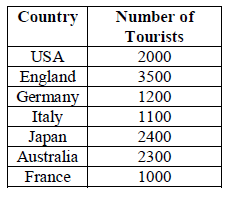Which two countries contributed to the one third of the total number of tourists who visited India in
2011?

Solution:
QUESTION: 9

If |−2x + 9| = 3 then the possible value of |−x| − x2would be:

Solution:
QUESTION: 10

All professors are researchers
Some scientists are professors
Which of the given conclusions is logically valid and is inferred from the above arguments:

Solution:
QUESTION: 11

Q. 11 – Q.35 carry one mark each.

Q.

The point group symmetry ofCH2=C=CH2 is

Solution:
QUESTION: 12

Two trial wave functions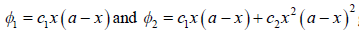give ground state
energies E1 and E2, respectively, for themicroscopic particle in a 1-D boxby using the variation
method. If the exact ground state energy is E0, the correct relationship between E0, E1 and E2 is

Solution:
*Answer can only contain numeric values
QUESTION: 13

The ground state energies of H atom and H2 molecule are –13.6 eV and –31.7 eV, respectively. The
dissociation energy of H2 is _______ eV.

Solution:
*Answer can only contain numeric values
QUESTION: 14

A 2 L vessel containing 2 g of H2gas at 27 °C is connected to a 2 L vessel containing 176 g of CO2
gas at 27 °C. Assuming ideal behavior of H2 and CO2, the partial pressure of H2 at equilibrium is
________ bar.

Solution:
QUESTION: 15

Consider the reaction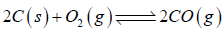at equilibrium.
The equilibrium can be shifted towards the forward direction by

Solution:
QUESTION: 16

A sparingly soluble electrolyte M2X ionizes as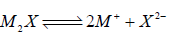The solubility product (Ksp), molal solubility (S) and mean molal activity coefficient (γ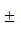) are related
by

Solution:
QUESTION: 17

For the first order consecutive reaction P → Q → R,
under steady state approximation to [Q], the variations of [P], [Q] and [R] with timeare best
represented by

Solution:
*Answer can only contain numeric values
QUESTION: 18

At 273 K and 10 bar,the Langmuir adsorption of a gas on a solid surface gave the fraction of
surface coverage as0.01. The Langmuir adsorption isotherm constant is _________ bar–1.
(Give the answer to the third decimal place)

Solution:
QUESTION: 19

Conversion of boron trifluoride to tetrafluoroborate accompanies

Solution:
QUESTION: 20

The correct statement with respect to the bonding of the ligands, Me3N and Me3P with the metal
ions Be2+ and Pd2+ is,

Solution:
QUESTION: 21

crystal has the lattice parameters a ≠ b ≠ c and α= β= γ= 90o. The crystal system is

Solution:
QUESTION: 22

The by-product formed in the characteristic reaction of (CO)5Cr=C(OMe)(Me) with MeNH2 is

Solution:
QUESTION: 23

The catalyst and co-catalyst used in the Wacker process, respectively, are

Solution:
QUESTION: 24

Oxymyoglobin Mb(O2) and oxyhemoglobinHb(O2)4, respectively, are

Solution:
*Answer can only contain numeric values
QUESTION: 25

Hapticity of cycloheptatrienein Mo(C7H8)(CO)3is _______.

Important : you should answer only the numeric value

Solution:
*Answer can only contain numeric values
QUESTION: 26

The number of oxygen molecule(s) that a molecule of hemerythrin can transport is _______.

Important : you should answer only the numeric value

Solution:
*Answer can only contain numeric values
QUESTION: 27

The maximum number of stereoisomers possible for the compound given below is _____.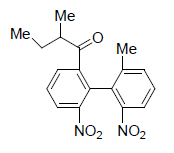Important : you should answer only the numeric value

Solution:
QUESTION: 28

The correct sequence of the amino acids present in the tripeptide given below is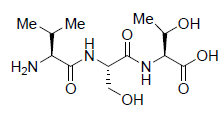Solution:
QUESTION: 29

Among the compounds given in the options A-D, the one that can be used as a formyl anion
equivalent (in the presence of a strong base) is

Solution:
QUESTION: 30

The major product formed in the reaction given below is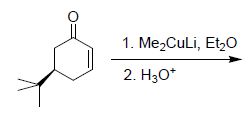Solution:
QUESTION: 31

The major product formed in the reaction given below is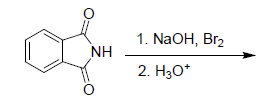Solution:
QUESTION: 32

The pericyclic reaction given below is an example of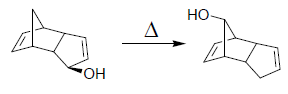Solution:
QUESTION: 33

The major product formed in the reaction of quinoline with potassium amide (KNH2) in liquid
ammonia is

Solution:
*Answer can only contain numeric values
QUESTION: 34

The number of signals that appear in the proton decoupled 13C NMR spectrum of benzonitrile
(C7H5N) is ________.

Solution:
QUESTION: 35

Among the compounds given in the options A-D,
the one that exhibits a sharp band at around 3300 cm–1 in the IR spectrum is

Solution:
*Answer can only contain numeric values
QUESTION: 36

Q. 36 to Q. 65 carry two marks each

Q.

In the metathesis reaction given below, 4.32 g of the compound X was treated with 822 mg of the
catalyst Y to yield 2.63 g of the product Z. The mol% of the catalystY used in this reaction is ___.
[Atomic weights of Ru = 101; P = 31; Cl = 35.5].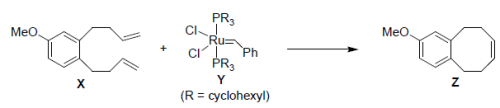Solution:
QUESTION: 37

An organic compound Q exhibited the following spectral data:
IR: 1760 cm-1
1H NMR: δ (ppm): 7.2 (1H, d, J = 16.0 Hz), 5.1 (1 H, m), 2.1 (3 H, s), 1.8 (3H, d, J = 7.0 Hz)
13C NMR: δ (ppm): 170 (carbonyl carbon).
Compound Q is

Solution:
QUESTION: 38

The major product formed in the Beckmann rearrangement of the compound given below is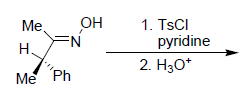Solution:
QUESTION: 39

The major product formed in the reaction given below is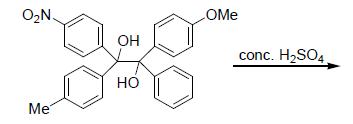Solution:
QUESTION: 40

The major product formed in the reaction given below is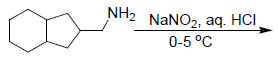Solution:
QUESTION: 41

The major product(s) formed in the reaction sequence given below is(are)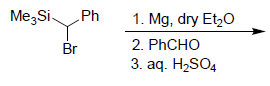Solution:
QUESTION: 42

Match the compounds in the column I with the photochemical reactions that they can undergo
given in the column II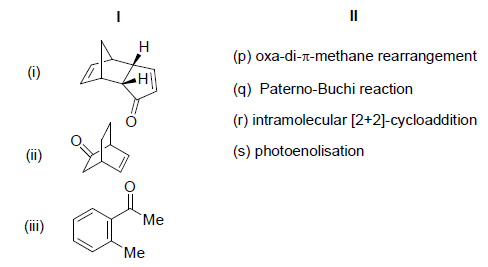Solution:
QUESTION: 43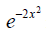is an eigen function of the operator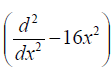. The corresponding eigen value is

Solution:
QUESTION: 44

The infrared spectrum of HCl gas shows an absorption band centered at 2885 cm–1. The zero point
energy of HCl molecule under harmonic oscillator approximation is

Solution:
QUESTION: 45

For the reaction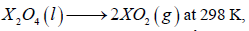given the values, ΔU = 9 kJ and ΔS = 84 J K–1, ΔG is

Solution:
*Answer can only contain numeric values
QUESTION: 46

The change in enthalpy when 3 mol of liquid benzene transforms to the vapor state at its boiling
temperature (80 °C) and at 1 bar pressure is _____________ kJ.

Solution:
*Answer can only contain numeric values
QUESTION: 47

The moment of inertia of a homonuclear diatomic molecule is 7.5 × 10–45 kg m2. Its rotational
partition function at 500 K is _____________ .

Solution:
QUESTION: 48

For a reaction of the type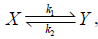the correct rate expression is

([X]0 and [X] correspond to the concentrations of X at time t=0 andt = t, respectively)

Solution:
QUESTION: 49

The temperature dependence of partition functions are as follows: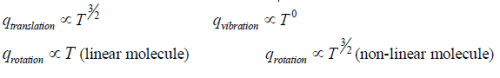According to the conventional transition state theory (CTST), the temperature dependence of the
Arrhenius pre-exponential factorfor a reaction of the type given below is

linear molecule + linear molecule ⇔ non-linear transition state →products

Solution:
QUESTION: 50

Decarbonylation reaction of [cis-(CH3CO)Mn(13CO)(CO)4] yields X,Y and Z, where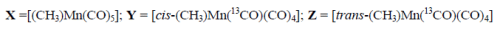The molar ratio ofthe products(X : Y : Z) in this reaction is

Solution:
QUESTION: 51

According to polyhedral electron count rule, the structure of Rh6(CO)16is

Solution:
QUESTION: 52

The increasing order of melting points of the halides NaCl, CuClandNaF is

Solution:
QUESTION: 53

The correct electronic configuration and spin only magnetic moment of Gd3+ (at.no. 64) are

Solution:
QUESTION: 54

Among the following octahedral complexes, the one that has the highest enthalpy of hydration is

Solution:
QUESTION: 55

A metal crystallizes in face-centered cubic lattice with a lattice parameter of 4.20 Å. The shortest
atom to atom contact distance in the lattice is

Solution:
QUESTION: 56

Polarographic method of analysis to obtain individual amounts of Cu2+ and Cd2+ in a given mixture
of the two ions (Cu2+ and Cd2+) is achieved by measuring their

Solution:
QUESTION: 57

The ground state term of [Ni(H2O)6]2+is

Solution:
QUESTION: 58

Common Data for Questions 58 and 59:

N,N-Dimethylformamide (DMF) gives different patterns of signals for the methyl protons whenits1H NMR spectrum is recorded at different temperatures.

Q.

Match the patterns of the NMR signals given in column I with temperatures given in the
column II.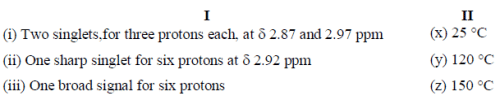Solution:
*Answer can only contain numeric values
QUESTION: 59

N,N-Dimethylformamide (DMF) gives different patterns of signals for the methyl protons
whenits1H NMR spectrum is recorded at different temperatures.

Q.

Based on the above data, the calculateddifference in the frequencies of the two methylsinglets, if
the spectrum is recorded on a 300 MHzspectrometer, is _________ Hz.

Solution:
QUESTION: 60

Common Data for Questions 60 and 61:

Heating a mixture of ammonium chloride and sodium tetrahydridoborate gives one liquid
product(X), along with other products, under ambient conditions.

Q.

Compound X is

Solution:
QUESTION: 61

Heating a mixture of ammonium chloride and sodium tetrahydridoborate gives one liquid
product(X), along with other products, under ambient conditions.

Q.

Compound X is an example of

Solution:
QUESTION: 62

The major product X formed in the reaction given below is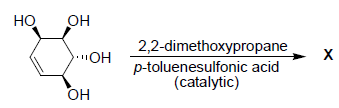Solution:
QUESTION: 63

Oxidation of the product X, obtained in the above reaction, with active manganese dioxide,
followed by acidic hydrolysis gives

Solution:
QUESTION: 64

The standard half-cell reduction potential of Fe3+ (aq) | Fe is – 0.036 V and that of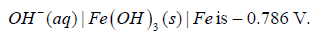Q.

For the determination of solubility product (KSP) of Fe(OH)3 ,
the appropriate cell representation and its emfare, respectively,

Solution:
QUESTION: 65

The standard half-cell reduction potential of Fe3+ (aq) | Fe is – 0.036 V and that ofQ.

The value of loge(KSP) for Fe(OH)3 at 298 K is

Solution:Use Code STAYHOME200 and get INR 200 additional OFF Use Coupon Code